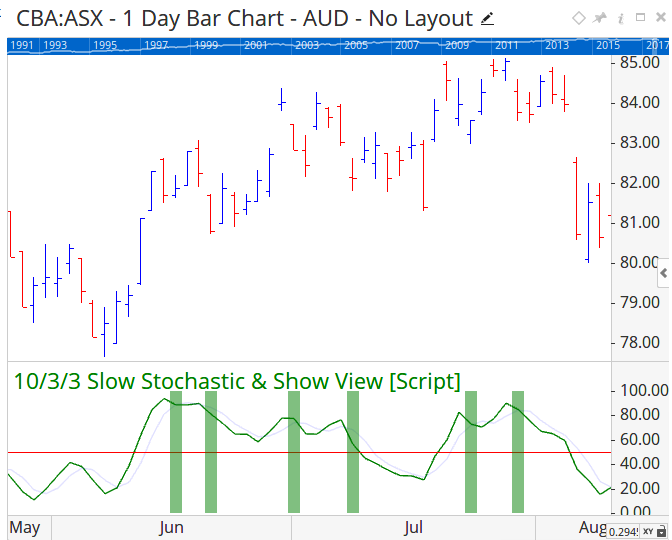# STOCHSCAN Function

Author: Optuma Team Last updated: Jul 14, 2020 05:47

## Overview

The STOCHSCAN function contains a number of preset criteria which can be used. So rather than having to build the criteria manually using several STOCH() functions, you can use a single function, which is faster.

STOCH().%D CrossesAbove STOCH().%K
You could use:

STOCHSCAN(SCANFOR=%K CrossesBelow %D) Matches
The available criteria are:

• %K CrossesAbove %D
• %K CrossesBelow %D
• %D CrossesAbove
• %D CrossesBelow
• %D TurnsUp - Below
• %D TurnsDown - Above
• %K CrossesAbove
• %K CrossesBelow
• %K TurnsUp - Below
• %K TurnsDown - Above

By default, on our stochastic indicators, the %K line is green and the %D line is blue. The STOCHSCAN function defualts to a Fast Stochastic, but can be changed to be Slow via the Type variable:

STOCHSCAN(TYPE=Slow)
The Matches operator turns the script into a Boolean result (True/False), which is required by the scanning manager. So, for the following example:

STOCHSCAN(TYPE=Slow, SCANFOR=%K TurnsDown - Above, VALUE=50.000) Matches
This would show a true result for any code where the Slow Stochastics %K line (green) turns down and its value is above 50.